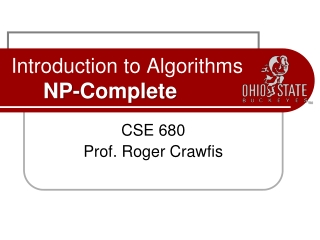DownloadDownload PresentationIntroduction to Algorithms NP-Complete

# Introduction to Algorithms NP-Complete

Download Presentation## Introduction to Algorithms NP-Complete

- - - - - - - - - - - - - - - - - - - - - - - - - - - E N D - - - - - - - - - - - - - - - - - - - - - - - - - - -
##### Presentation Transcript

1. Introduction to AlgorithmsNP-Complete CSE 680 Prof. Roger Crawfis

2. Polynomial Time • Most (but not all) of the algorithms we have studied so far are easy, in that they can be solved in polynomial time, be it linear, quadratic, cubic, etc. • Cubic may not sound very fast, and isn’t when compared to linear, but compared to exponential we have seen that it has a much better asymptotic behavior. • There are many algorithms which are not polynomial. In general, if the space of possible solutions grows exponentially as n increases, then we should not hope for a polynomial time algorithm. These are the exception rather than the rule.

3. Hard Problems • Problems with no known polynomial solution are called Hard problems. • Another class of problems (NP) seem like they should be easy. They have the following property: • A solution can be verified in polynomial time. • Consider the following very common example called Satisfiability: (SAT) • Input: A Boolean expression, F, over n variables (x1,. ..,xn) • Output:1 if the variables of F can be fixed to values which make F equal true; 0 otherwise.

4. NP Problems • NP stands for non-deterministic polynomial time. • The basic premise is we could guess at a solution and then test whether we guessed right or not easily. Guessing is of course non-deterministic! • So, think of this as guessing in polynomial time. No guarantee that you will ever guess correctly though.

5. Polynomial Time Reductions • The SAT problem is a circuit, so it is very useful and interesting. Can we write a program to do X? This and many other problems have been studied at length. • There are many mappings (called reductions) that have been discovered that map one problem to the other, so if we solve one we can solve the other. • Moreover, these mappings are polynomial time mappings.

6. NP-Complete • The set of NP problems that can be mapped to each other in polynomial time is called NP-Complete. • It is difficult to prove that something can not be done. • Let me repeat that, it is difficult to prove that something can not be done. • So, while we know how to solve many of these algorithms in exponential time, whose to say that one of you bright students won’t come up with a clever polynomial time algorithm! • NP-Complete is useful from that standpoint, if any of them turns out to have a polynomial time algorithm, they all do (hence P=NP). • Of course, us old professors feel that these problems have been looked at from every angle and no such solution will ever be found (hence, P ≠ NP).

7. NP-Complete • It is also useful to know these algorithms, as they occur frequently in real applications and tackling them in a brute force fashion may be disastrous. • SAT problem • Traveling Salesman problem • Knapsack Problem • Longest Path • Graph Clique

8. TSP • For each two cities, an integer cost is given to travel from one of the two cities to the other. The salesperson wants to make a minimum cost circuit visiting each city exactly once. 2 i = 23 2 1 1 3 4 1 3 4 5 4 2 1 2 2 2 2 1 1 1

9. Circuit-SAT • Take a Boolean circuit with a single output node and ask whether there is an assignment of values to the circuit’s inputs so that the output is “1” Logic Gates 0 1 0 1 1 NOT 1 1 0 OR 1 0 1 0 1 AND

10. Knapsack • Given s and w can we translate a subset of rectangles to have their bottom edges on L so that the total area of the rectangles touching L is at least w? 5 6 2 3 4 7 1 L s

11. UnweightedBipartite Matching

12. Definitions Matching Free Vertex

13. Definitions • Maximum Matching: matching with the largest number of edges

14. Definition • Note that maximum matching is not unique.

15. Intuition • Let the top set of vertices be men • Let the bottom set of vertices be women • Suppose each edge represents a pair of man and woman who like each other • Maximum matching tries to maximize the number of couples!

16. Graph Clique • Set of verticessuch that theyall connectto eachother.

17. K-Cliques • A K-clique is a set of K nodes with all K(K-1)/2 possible edges between them This graph contains a 4-clique

18. K-Cliques • Given: (G, k)Question: Does G contain a k-clique? • BRUTE FORCE: • Try out all {n choose k} possible locations for the k clique

19. NP-Complete • It is also interesting to look at the relationship between some easy problems and hard ones: